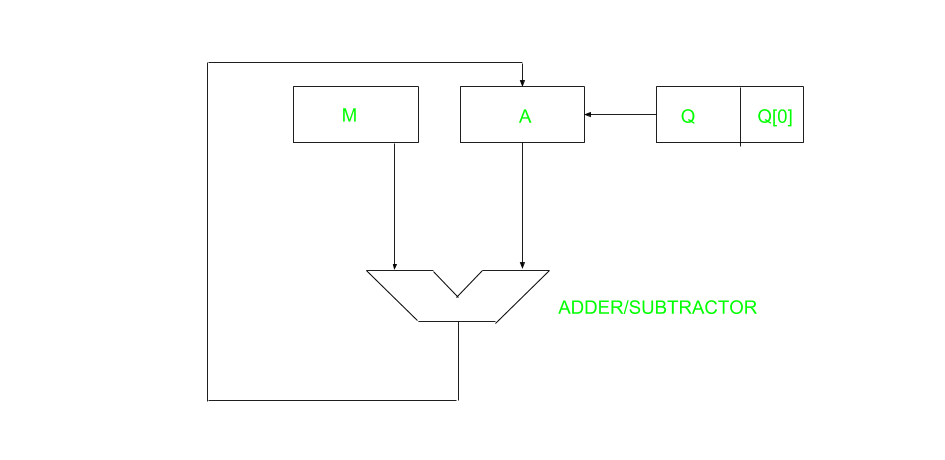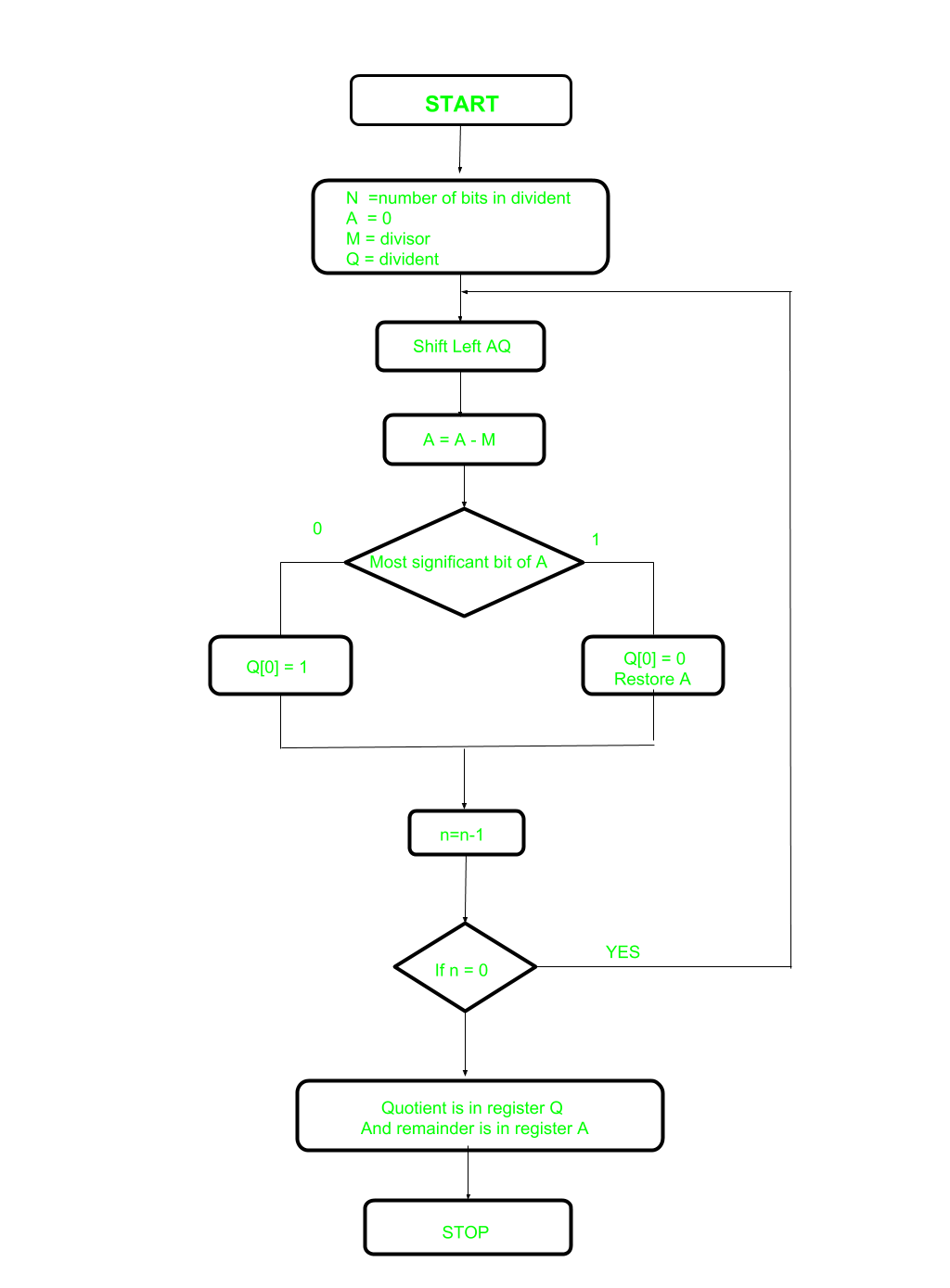Related Articles
Restoring Division Algorithm For Unsigned Integer
• Difficulty Level : Easy
• Last Updated : 22 Apr, 2020

A division algorithm provides a quotient and a remainder when we divide two number. They are generally of two type slow algorithm and fast algorithm. Slow division algorithm are restoring, non-restoring, non-performing restoring, SRT algorithm and under fast comes Newton–Raphson and Goldschmidt.

In this article, will be performing restoring algorithm for unsigned integer. Restoring term is due to fact that value of register A is restored after each iteration.Here, register Q contain quotient and register A contain remainder. Here, n-bit dividend is loaded in Q and divisor is loaded in M. Value of Register is initially kept 0 and this is the register whose value is restored during iteration due to which it is named Restoring.Let’s pick the step involved:

• Step-1: First the registers are initialized with corresponding values (Q = Dividend, M = Divisor, A = 0, n = number of bits in dividend)
• Step-2: Then the content of register A and Q is shifted left as if they are a single unit
• Step-3: Then content of register M is subtracted from A and result is stored in A
• Step-4: Then the most significant bit of the A is checked if it is 0 the least significant bit of Q is set to 1 otherwise if it is 1 the least significant bit of Q is set to 0 and value of register A is restored i.e the value of A before the subtraction with M
• Step-5: The value of counter n is decremented
• Step-6: If the value of n becomes zero we get of the loop otherwise we repeat from step 2
• Step-7: Finally, the register Q contain the quotient and A contain remainder

Examples:

```Perform Division Restoring Algorithm
Dividend = 11
Divisor  = 3```
nMAQOperation
400011000001011initialize
0001100001011_shift left AQ
0001111110011_A=A-M
00011000010110Q=0 And restore A
30001100010110_shift left AQ
0001111111110_A=A-M
00011000101100Q=0
20001100101100_shift left AQ
0001100010100_A=A-M
00011000101001Q=1
10001100101001_shift left AQ
0001100010001_A=A-M
00011000100011Q=1

Remember to restore the value of A most significant bit of A is 1. As that register Q contain the quotient, i.e. 3 and register A contain remainder 2.

My Personal Notes arrow_drop_up